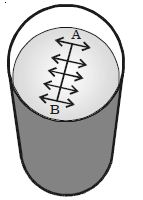# Surface Tension of a Liquid

Surface tension is the property of the free surface of a liquid at rest to behave like a stretched membrane in order to acquire minimum surface area.

Imagine a line AB in the free surface of a liquid at rest (Figure). The force of surface tension is measured as the force acting per unit length on either side of this imaginary line AB. The force is perpendicular to the line and tangential to the liquid surface. If F is the force acting on the length 1 of the line AB, then surface tension is given by: T = F/lSurface tension is defined as the force per unit length acting perpendicular on an imaginary line drawn on the liquid surface, tending to pull the surface apart along the line. Its unit is N m-1 and dimensional formula is MT-2.